# University of California, San Diego Physics 11 - Survey of Physics

 H. E. SmithPhysics 11 - The Units of ScienceIntroduction

It's always a good idea when you're traveling, to try and learn a few words of the local language. So, as you set out to explore the physical world, let me introduce you to a few words, units and "currency" that you'll encounter on the trip.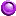Scientific Notation

The important numbers in Physics span almost 40 orders of magnitude in size. Consider the mass of the Sun:

MSun = 1,989,000,000,000,000,000,000,000,000,000 kilograms

 It's cumbersome, to say the least, having to write out all of those zeros. Even changing the units to metric tons (eliminate 3 zeros) doesn't help much. Furthermore, we really don't know the Sun's mass beyond the accuracy of the fourth digit. All those zeros are just place-keepers, carrying no useful information. For this reason, scientists use a short-hand called scientific notation to express very large or very small numbers. In scientific notation the Sun's mass becomes: MSun = 1.989 x 10 30 kg.The number above the ten, called the power of ten or exponent, stands for the number of decimal places. If it is positive, as in the mass of the Sun, the decimal places are in front of the decimal point. So, 1030 means "move the decimal point 30 places to the right and fill the empty places with zeros" (or, more mathematically, multiply by ten 30 times).

For very small numbers, such as the mass of the proton, we use negative powers of 10.

Mp+ = 0.000000000000000000000000001673 kilograms

In scientific notation, mass of the proton becomes
Mp+ = 1.673 x 10 -27 kg.

For negative exponents, the powers of 10 are after the decimal point; 10-27 means "move the decimal point 27 places to the left and fill in with zeros" (or divide by ten 27 times).

There are several good web pages about Scientific Notation. If you would like to read a bit more, try out the University of Maryland's Astronomy Programs site, with a Scientific Notation Exercise and an Astronomical Distance Calculator.

###Arithmetic in Scientific Notation

Arithmetic with scientific notation is just two easy steps, as shown in the tables below.

Multiplication
Step 1: Multiply numbers

So:

(5 x 10 15) multiplied by (3 x 10 4) = (5 x 3) x 10 (15 + 4) = 15 x 1019

For addition or subtraction, you have to be careful to convert to the same exponent.

Step 1: Convert to a common exponent

2.5 x 10-2 added to 3.1 x 10-3= 0.025 + 0.0031 = 0.0281 (or 2.81 x 10-2 = 28.1 x 10-3)

Let's try an example. Suppose you want to estimate the mass of our Galaxy, the Milky Way.
In round numbers, there are about a half-trillion stars in the galaxy.

Number of stars = N*5 x 1011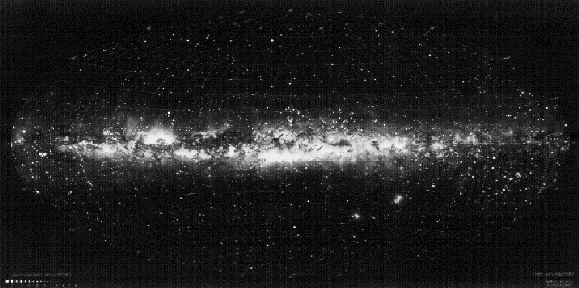The Milky Way Galaxy - count the stars if you have a bit of free time.

The mass of the sun, a typical star is about 2 x 1030 kilograms. (Your mass is about 70 kgs!)

Typical star mass = M*2 x 1030kilograms

Then, the mass of the Milky Way is the number of stars times the mass of a typical star:

MMWN* x M*(5 x 1011stars) x (2 x 1030kgs)

So that:

MMW( 5 x 2 ) x 10 (11 + 30) kilograms10 x 1041 kg = 1 x 1042 kg.

This is, of course, a crude estimate, especially because we now know that the mass of the Milky Way is dominated by unseen matter.

Division in Scientific Notation is just the inverse process:

Division
Step 1: Divide numbers
Step 2: Subtract exponents

Another example will show how it's done. Suppose you wanted to estimate the number hydrogen atoms in the sun. One estimate would be to divide the sun's mass by the mass of a hydrogen atom. (This assumes that the Sun is composed entirely of hydrogen atoms. It is mostly hydrogen, with some helium and other heavier elements thrown in.)

NHMsun / mH2 x 1030kg / 1.67 x 10-27kg

Now we divide the 2 and the 1.67 (Again this is a crude estimate so we can take mH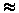2 x 10-27kg) and subtract the exponent of the divisor (-27) from the exponent of the dividend (30).

NH(2 / 2) x 10(30-[-27])1057

If you are very careful you can verify these calculations by longhand.

##Units of Measurement

Before people can share information about the physical world, they need a common language and standard units of measurement. Since science is an international human endevour, scientists all over the world have agreed to use one set of units when they talk with each other about their work. This world-wide standard is called the metric system . So, when Scientist A in Katmandu says the distance to the Sun is 150,000,000 kilometers, Scientist B in Helsinki knows exactly what that means. For a nice introduction to units of measurement, see this site.
Here is a list of fundamental units from the National Institute of Standards and Technology (NIST), formerly the National Bureau of Standards.

The metric system has standard prefixes to indicate relative sizes. As we noted above, Scientist A reported that the distance to the Sun was 150,000,000 kilometers. The prefix "kilo" means 1,000, so a kilo meter is 1,000 meters. The table below gives some metric names used in these pages and their definitions.

Metric Prefixes
PREFIX DEFINITION SCIENTIFIC
NOTATION
PREFIX DEFINITION SCIENTIFIC
NOTATION
tera 1,000,000,000,000 1012 centi .01 10-2
giga 1,000,000,000 109 milli .001 10-3
mega 1,000,000 106 micro .000001 10-6
kilo 1,000 103 nano .000000001 10-9
deka 10 101 pico .000000000001 10-12
deci .1 10-1 femto .000000000000001 10-15

Here is a list of prefixes from the National Institute of Standards and Techonology (NIST).

A few examples of how to use the metric prefixes:

10-2 pedes = 1 centipede
10-3 vanillis = 1 millivanilli
10-12 boos = 1 picoboo
10-15 bismols = 1 femtobismol

Even the "world standard" metric system has different versions. Astronomers use a version
of the cgs (Centimeter-Gram-Second) system, modified for the immense distances in the Universe and huge masses of astronomical objects. Most physicists are converging on use of the MKS (Meter-Kilogram-Second) version or Systeme Internationale (SI).Length

To describe distances and sizes, we define a standard of length. The SI unit of length is the meter, abbreviated "m". A meter is a little longer than a yard (39.37 inches)

There are three special units of distance used by astronomers. These are the astronomical unit (AU), the light-year and the parsec. The astronomical unit is the average distance of the Earth from the Sun shown above.

1 AU = 1.5 x 1010 m = 150 million km = 93 million miles = 8.3 "light-minutes"

A light-year (ly) sounds like a measure of time, but it is a length - the distance light travels in one year.(We can use a light-year as a unit of measure because ALL light travels at the same speed; it is a fundamental constant of the Universe. More about this later...) So, in one year, light travels:The name parsec comes from the technique of measuring distance called parallax. The nearest star, Alpha Centauri, is about 1.3 pc or 4 light-years away.

1 parsec = 3.26 light-years

In addition to these distance units, astronomers use the Ångstrom(Å) as a measure of size on the atomic scale.

1 Ångstrom = 10-10 m.

An Ångstrom is about the size of a hydrogen atom. Optical astronomers use the Ångstrom to measure wavelengths of light. The nanometer (nm = 10-9m = 10Å) is also used as a measure of optical light wavelength, and the micrometer or micron ( µm = 10-6m = 103nm = 10,000Å) is used to describe infrared wavelengths.Mass

Everyone knows that the astronauts weigh less when they're walking on the Moon than when they're back on Earth. Since the Moon is less massive than the Earth, it's gravitational attraction is smaller. It is essential to have a unit for measuring "amount of stuff" that would be the same everywhere in the Universe. That unit of "stuff" is called mass. So, an astronaut's weight is less on the Moon, but his/her mass is exactly the same. Actually,weight and mass are two different things. Your weight is the gravitational attraction between you and the Earth. (Or whatever planet you may be visiting.) Your mass is a measure of your inertia, your resistance to changes in motion. Here is a nice explanation of mass.

The SI unit of mass is the kilogram. It is about 10% over two pounds:

0.454 kg = 454 grams = 16 ounces = 1 pound

The defining standard of mass is a 1-kilogram platinum-iridium cylinder located in the International Bureau of Weights and Measures in Sevres,France. There is a copy of the standard in the National Institute of Standards and Technology in Boulder, and other national laboratories around the world.
Should the international standard of the kilogram be redefined? Read about it here.

On the very large (astronomical) scale and the very small (atomic) scale two other units of mass are used. To measure atomic masses the atomic mass unit (amu) is employed. the amu is defined as one-twelfth the mass of a common carbon atom:

1 amu = m(12C)/12 = 1.66 x 10-27kg

slightly less than the mass of a proton. For stars, galaxies, etc. we use the solar-mass

M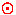2 x 1030kg

where the "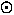" is the standard astronomical symbol for the sun. The mass of our Milky Way Galaxy is about 1012M.Time

 The MKS unit of time is the second. The time standard is kept by the NIST using a cesium atomic clock like the one to the right. Visit the Atomic Clock tour exhibit. Synchronize your watch to "official" US time.Atomic clock at NISTEnergy

Much of physics and astrophysics is concerned with understanding the energy generation and energy output from objects. Energy conservation principles are among the most important and useful physical tools. The SI unit of energy is the joule. A joule is the amount of energy contained in the motion of a 1 kilogram mass moving with a velocity of 1 meter/second or about the energy represented by a brick moving at 2 mph. The energy output, production, or consumption of an object is its Power, measured in joules/second or Watts. A 100-watt light bulb consumes 100W of electrical energy. A possibly more familiar unit of power, the horsepower is related by:

At the atomic / nuclear level, energies are often given in electron-volts (abbreviated as "eV"), the energy of an electron accelerated through a voltage of 1 volt (1 eV = 1.6 x 10-19 joules). The energy-levels and ionization energies of most atoms are a few eV. X-ray photon energies are frequently quoted in kilo-electron-volts(KeV). Gamma-rays and nuclear energies are most often in MeV.

As stated in Einstein's formula,

E=mc2,

mass and energy are the same; one can be converted into the other. For this reason, the masses of subatomic particles like protons and electrons are sometimes given as the equivalent energy, usually in MeV. This is also called the "rest-mass energy" of the particle. The "rest-mass energy" of the electron,m e = 0.511 MeV. The proton "rest-mass energy", mp = 938 MeV. The accelerators or "atom-smashers" used by particle physicists require these enormous energies for production of elementary particles.Force

In nature, forces are the "pushes" that cause masses to move.F = ma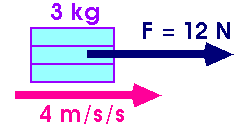Newton's Second Law states that force equals mass times acceleration. We discussed units of mass earlier. Acceleration is a change in the velocity an object is moving, either increase or decrease. (Acceleration is positive if the velocity of an object is increasing, and acceleration is negative if the object is slowing down.) So, forces cause a change in the velocity of an object. That means that an object at rest (velocity = 0), or an object moving with constant (unchanging) velocity is not being acted upon by an forces. No change in velocity means no forces or a balance of forces that cancel each other out.

We started this discussion of physical quantities and their units of measurement, because we wanted a common frame of reference that would be understood and accepted by scientists anywhere in the world. We now need some units for describing forces and accelerations. But, suddenly, we need units that are different than any we have used so far. If I want to tell you about the velocity or acceleration of a rocket, there are 2 parts to the description. I must tell you how fast the speed is changing, AND in in what direction it is going. The units of mass and energy that we encountered earlier had only one part. Even units of time have only one part, because, for us, time flows in one direction only. But, physical objects can move in any direction and can speed up or slow down. So the units of force, velocity, and acceleration must indicate the "amount of change" and the "direction of motion". Quantities with both magnitude and direction are called vectors. Physical quantities that have only magnitude, like mass or energy, are called scalars.

• A good introduction to vectors.
• More about vectors with self-test.

In the SI system, the unit of force is the Newton .

1 Newton = 1 kilogram-m/sec2

•Conducted by Gene Smith, CASS/UCSD.
Comments? You may send email to hsmith@ucsd.edu

Prof. H. E. (Gene) Smith
CASS   0424   UCSD
9500 Gilman Drive
La Jolla, CA    92093-0424

Last updated: 25 Sept 2003